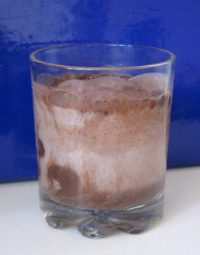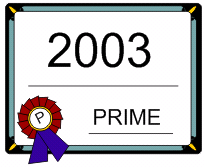A question: Is math well mixed?

User Rating:/ 1One can build math starting from a simple original ideas. In terms of Kolgomorov complexity ( EN ) the "program for making math" is simple. The same appears when you put cocoa powder into milk. Just two ingredients in the beginning. When mixed a little it is an interesting fight between cocoa and milk. But quickly it will be well mixed and everything interesting disappears.

A question: Is math well mixed?

A nice discussion about the complexity and complexodynamics can be found in Scott Aaronson's Shtetl-Optimized blog ( EN , EN ).

PRIMES is in P. Where in P?

User Rating:/ 1

..

In 2002-2003 Agrawal, Kayal, and Saxena in a great breakthrough "PRIMES is in P"   ( Wiki EN ) proved that the PRIMES function (prime number test)  is O(log ^8 n), in other words polynomial of degree 8 of the number of digits (log n) of a given number n.

CongratulationsBTW, where in P is its real position? Any idea?

Scottish Book *****

User Rating:/ 1

Problem book of Polish mathematicians discussing in the  Scottish Café  (1930s and 1940s, Lwów, wiki EN ).

Full texts ( manuscript PL and typescript EN ).

Hilbert's thirteenth problem ***

User Rating:/ 0

Hilbert (1900) : Is it possible to create a function of several variables using functions of two variables? ( EN )

Arnold (1957, nineteen years old) : Yes.